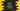# Python program to count the total number of lines in a file## Python program to count the total number of lines in a file :

In this tutorial, we will learn how to find the total number of lines in a file using python. Actually, we will find out the total lines in a text file. With this tutorial, you will learn how to open a file and read its content in python. Python provides default methods to read, write, and delete a file. You can use these file operations out-of-the-box without importing any external module.

We are using one hard-coded file name in the program. But you can also get the file name from the user. The algorithm we are using in this program is as below :

## Algorithm :

1. Open the file in read mode. For reading a file, we need to open it first. We are opening the file in read mode as we are only reading the content of the file.
2. Initialize one counter as 0. This counter will hold the total number of lines in the file.
3. Using a for loop, read the file line by line.
4. Increment the counter by 1 for each line. Keep the counter increasing until the file reading is completed. The counter will hold the total number of lines at the end.
5. Finally, print out the counter, i.e. the total number of lines.

### Python program :

``````#1

#2
lines_count = 0

#3
with open(file_path,'r') as f:
#4
for l in f:
#5
lines_count = lines_count +1

#6
print("Total number of lines : ",lines_count)``````

The code is shared here on Github.

### Explanation :

The commented numbers in the above program denote the step-number below :

1. Store the file path in the variable filepath_. Change the file path to the file you want to check.
2. Initialize one variable linescount_ as 0. This variable will save the count of total lines in the above file.
3. Open the file in “read” mode or r. open method takes two arguments. The first argument is the file that we want to open and the second argument is the kind of permission that we want for opening the file. Here, we are opening the file in read mode, so ‘r’ string is passed as the second argument. If you want to write anything to the file, you will have to pass ‘w’ or if you want to append anything to the file, you will have to pass ‘a’ as the second argument.
4. Using one for loop, read line by line. We have opened the file as ‘f’,  i.e. the variable ‘f’ will hold the content of the file we opened. The for loop is reading all lines in that variable, or in that file.
5. For each line, increment the value of linescount_ by 1. As explained above, this counter is increased by one on each step of the loop. When the loop will exit, this variable will hold the total count of lines in the file.
6. Finally, print out the total number of lines i.e. linescount_.

### Example Output :

```Total number of lines :  7```

### Conclusion :

In this tutorial, we have learned how to count the total number of lines of a file in python. We have learned how to open a file and how to read its content. Try to run the example above and drop one comment if you have any queries.